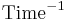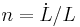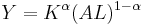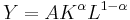Solow–Swan model

The Solow–Swan model is a long-run economic growth model.

Model assumptions

• single-sector economy
• no taxation

etc.

Variables in the model

Name Variable Unit Set of possible values Rival input? Variable type Notes
Output Y Units of GDP (dollar?) [0, ∞) Endogenous
Physical capital (capital stock) K [0, ∞) Yes Endogenous Physical capital includes things like machines, computers, buildings, etc.
Labor L [0, ∞) Yes Exogenous
Technology (knowledge) A, T No Exogenous
Consumption C
Investment I
Amount saved S
Growth of X$g_X = \dot{X}/X = \frac{\frac{\partial X}{\partial t}}{X}$$\text{Time}^{-1}$
Population growth$n = \dot L / L$$\text{Time}^{-1}$ (−∞, ∞)
Depreciation (rate?) δ, d, D Unitless
Capital per worker k = K/L Endogenous
Fraction saved s Unitless [0, 1]
Output per worker y = Y/L Endogenous
Time t Time, e.g. years
Production function F
Elasticity of output with respect to capital α Unitless (0, 1)

Mathematical formalism$Y = K^\alpha (AL)^{1-\alpha}$ (sometimes also$Y = AK^\alpha L^{1-\alpha}$)$\dot K = sY - \delta K$

TODO show that the model satisfies (1) constant returns to scale; (2) diminishing returns to capital; (3) diminishing returns to labor; (4) the Inada conditions.

A closed form is possible, but it is possible to play around with the model in non-closed forms to extract useful information.